### Step 1: Load the Data

We will use the airquality data set, which you should already have as part of your R installation.

``````  # Assign the built-in "airquality" dataset into a new variable called "air"
air <- data.frame(airquality)``````

### Step 2: Clean the data

After you load the data, there will be some NAs in the data. You need to figure out what to do about those nasty NAs.

`````` # find which columns in the dataframe contain NAs.
colnames(air)[colSums(is.na(air)) > 0]``````
``##  "Ozone"   "Solar.R"``
`````` # Check if the NAs in column "Ozone" and replace them by the mean value of this column
air\$Ozone[is.na(air\$Ozone)] <- mean(air\$Ozone, na.rm=TRUE)
# Check if the NAs in column "Solar.R" and replace those NAs by the mean value of this column
air\$Solar.R[is.na(air\$Solar.R)] <- mean(air\$Solar.R, na.rm=TRUE)``````

### Step 3: Understand the data distribution

Create the following visualizations using ggplot:

• Histograms for each of the variables
• Boxplot for Ozone
• Boxplot for wind values (round the wind to get a good number of “buckets”)
``````  # histograms for each of the variables
# use the Ozone in "air" dataframe as X variable to create a histogram
# set the bin width to be 5, border color to be white, and bin color to be black
ggplot(air, aes(x=Ozone)) + geom_histogram(binwidth=5, color="white", fill="black")``````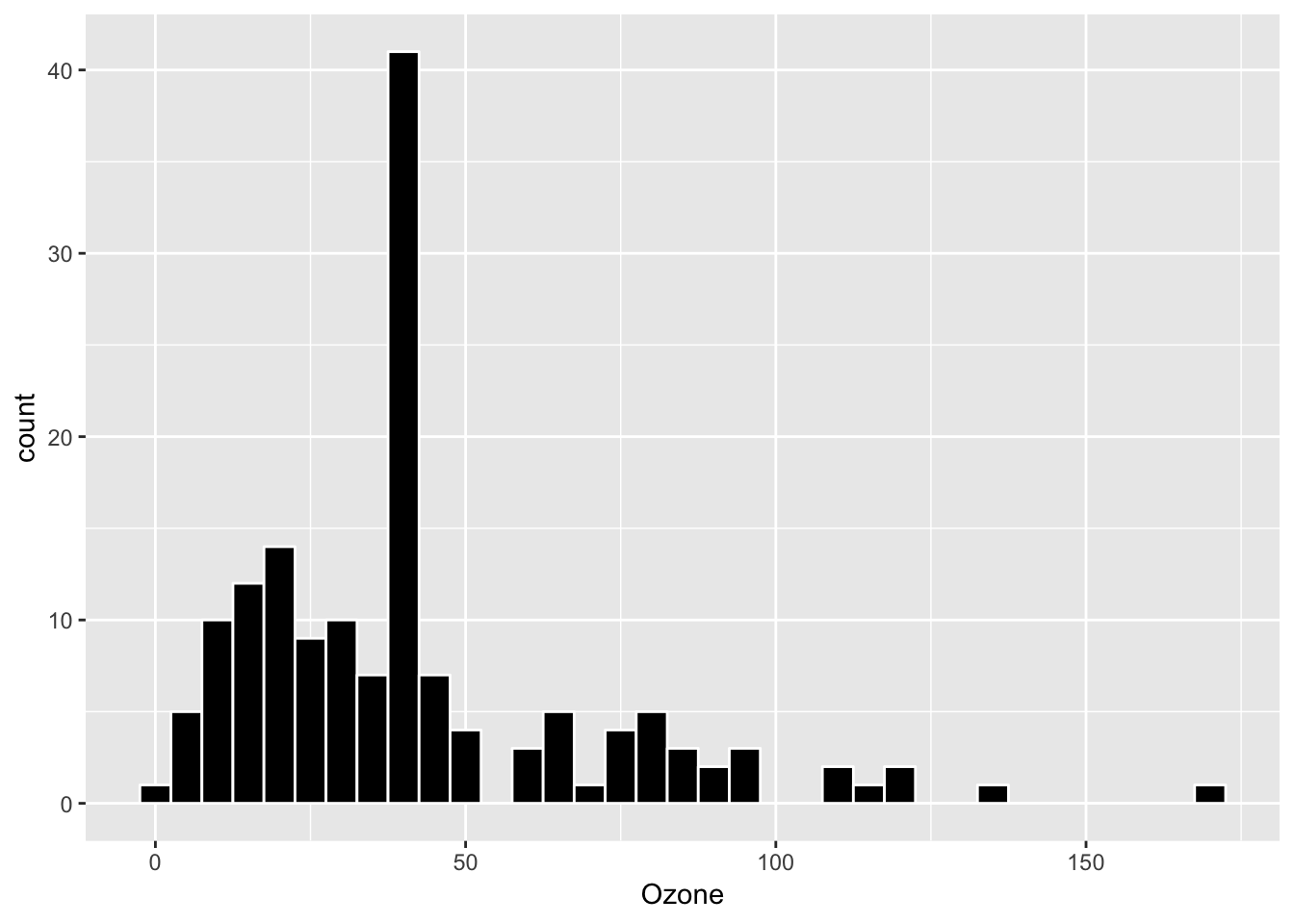``````# use the Solar in "air" dataframe as X variable to create a histogram
ggplot(air, aes(x=Solar.R)) + geom_histogram(binwidth=5, color="white", fill="black")``````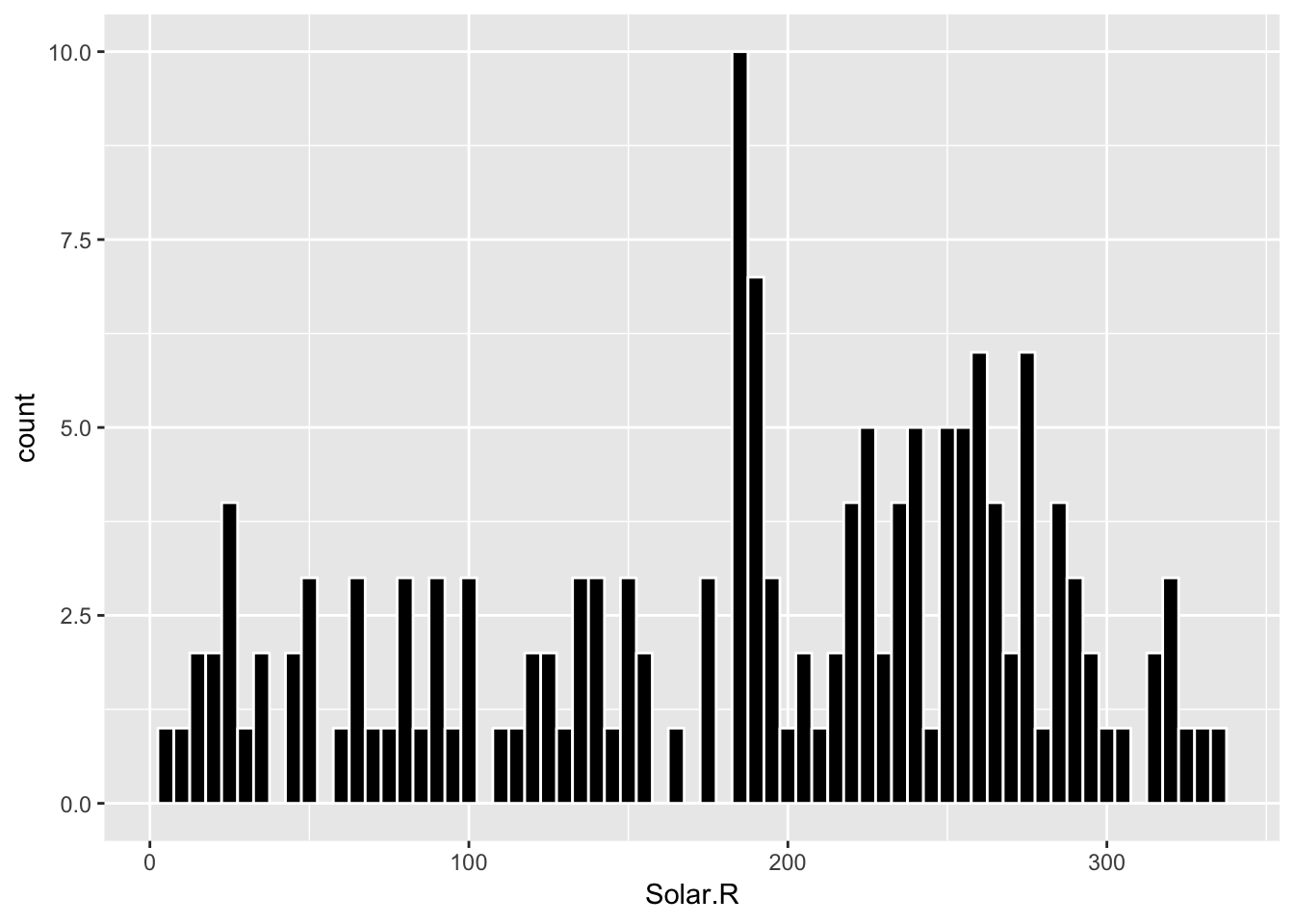`````` # use the Wind in "air" dataframe as X variable to create a histogram
ggplot(air, aes(x=Wind)) + geom_histogram(binwidth=0.5, color="white", fill="black")``````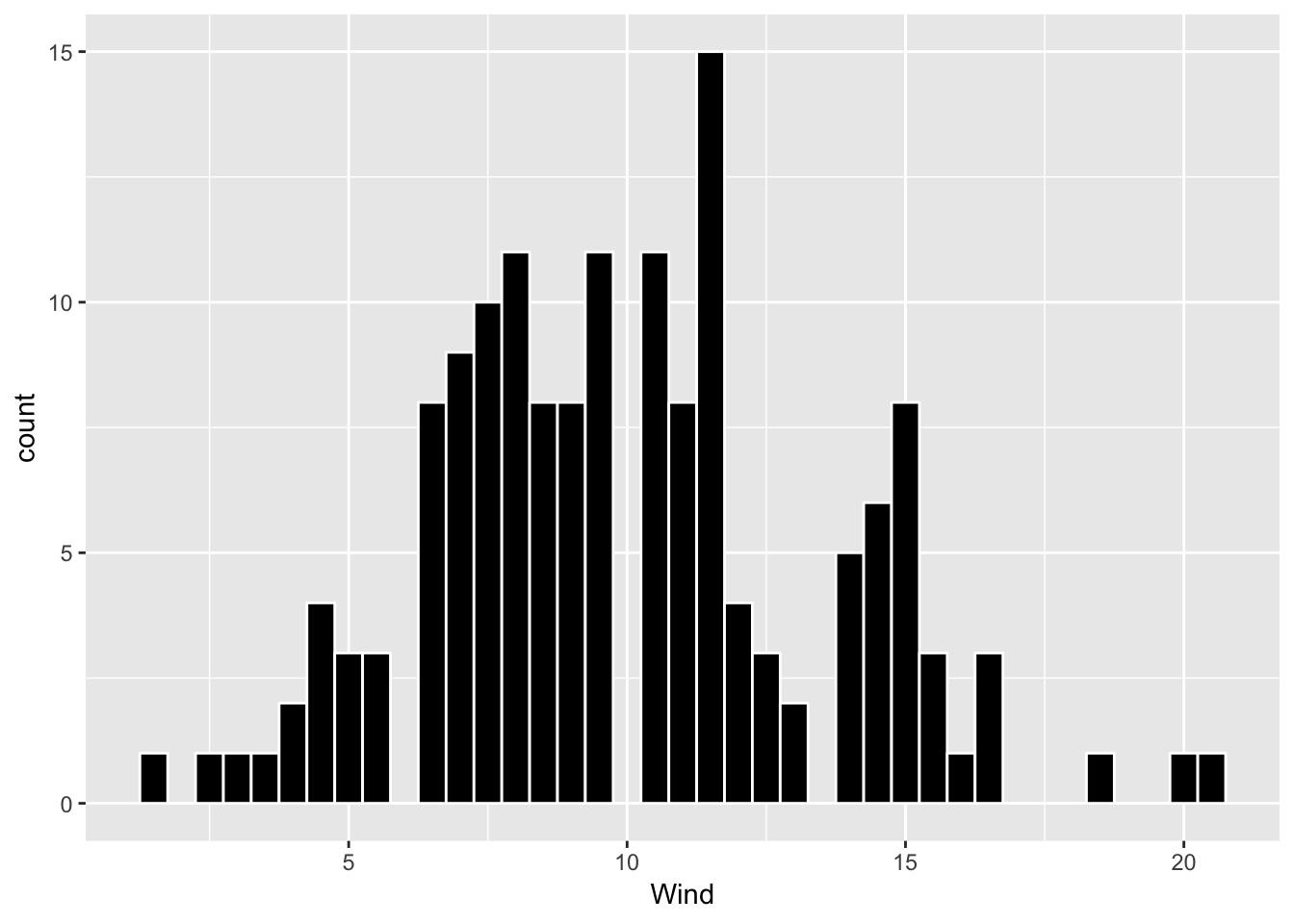``````  # use the Temp in "air" dataframe as X variable to create a histogram
ggplot(air, aes(x=Temp)) + geom_histogram(binwidth=2, color="white", fill="black")``````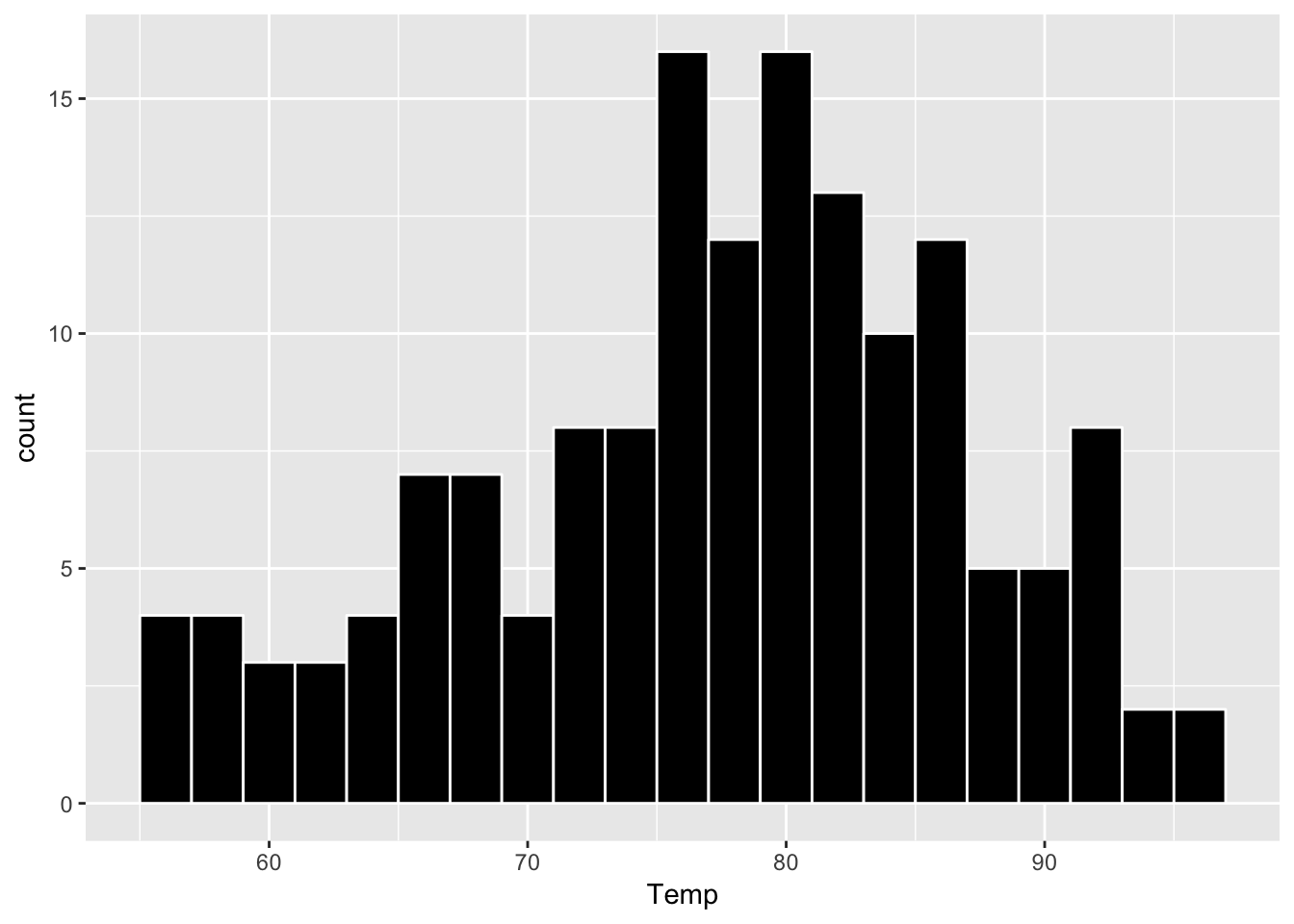``````  # use the Month in "air" dataframe as X variable to create a histogram
ggplot(air, aes(x=Month)) + geom_histogram(binwidth=1, color="white", fill="black")``````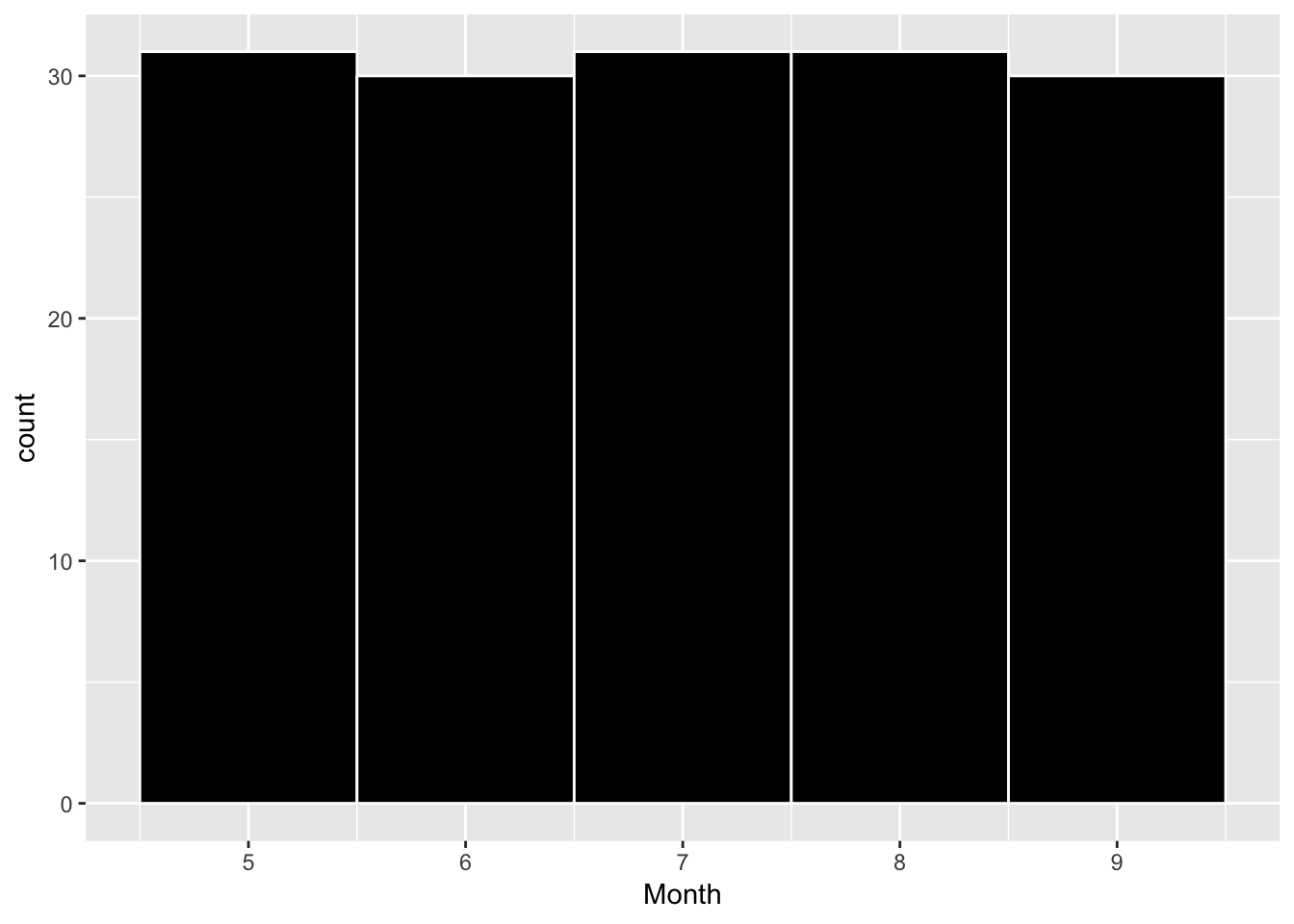``````  # use the Day in "air" dataframe as X variable to create a histogram
ggplot(air, aes(x=Day)) + geom_histogram(binwidth=1, color="white", fill="black")``````